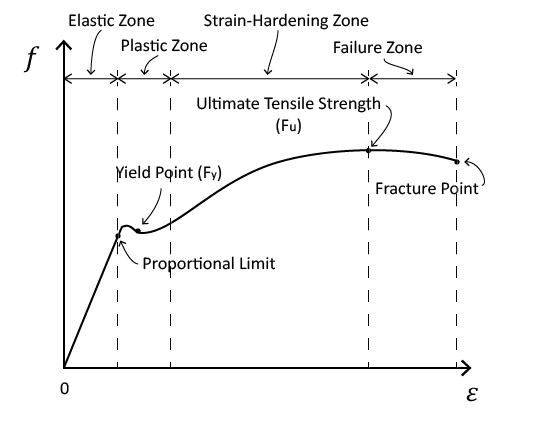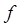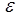Fundamentals Modulus of Elasticity (Young's Modulus)Slope of the stress-strain diagram within the elastic zone is called “Modulus of Elasticity” or “Young’s Modulus”. This parameter depends only on the material type.Stress-Strain Diagram In this equation E is the “Modulus of Elasticity”,is the applied stress in psi or ksi within the elastic limit, andis the corresponding strain. Since strain does not have any units, E has units of psi or ksi. For structural steel E29,000 ksi. A smaller modulus of elasticity translates to more a flexible (or less rigid) member.© 2011 Virginia Polytechnic Institute and State University